# How To Concatenate Cells If Same Value Exists in Another Column in Excel

This post will guide you how to concatenate cells if same value in another column using an VBA macro in Excel. How do I merge cells if same value exists in another column using formula and filter feature in Excel 2013/2016.

Assuming that you have a list of data in range A1:B6, you wish to concatenate cells in column B if the approatiate cell values are same in the column A. How to do it. And you can use an VBA Macro to quickly concatenate cells based on your conditaion. or you can use another method based on Formulas and filter function.

### Concatenate Cells If Same value Exists in Another Column using Formulas

To concatenate cells in range B1:B6 based on the cell values in range A1:A6 if they are same, you can use two formulas based on the `IF function` and the `Concatenate function`. Just do the following steps:

#1 select one blank cell (Cell c2) beside range B1:B6, and then type the following formula, press `Enter` key on your keyboard to apply it.

`=IF(A2<>A1,B2,C1 & "," & B2)`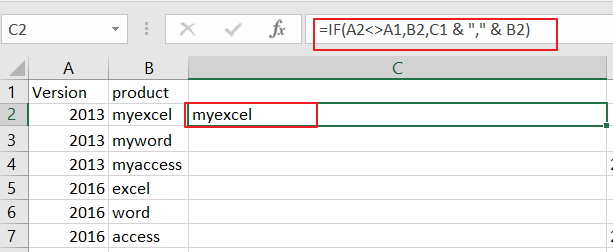#2 drag the AutoFill Handle in cell C2 down to other cells to apply this formula.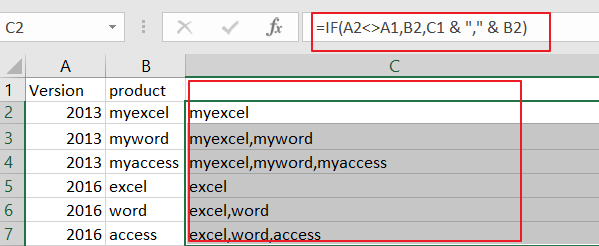#3 you need to type anothe formula in Cell D2, then press `Enter` key.

`=IF(A2<>A3,CONCATENATE(A2,",",C2),"")`

#4 drag the AutoFill Handle in cell D2 down to other cells to apply this formula. You would see that the cell values in range B1:B6 have been concatenated in range D1:D6.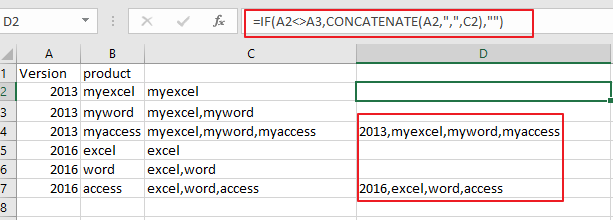#5 Now you need to filter out all blank cells in range D1:D6. And select all cell in Range D1:D6, and go to `Data` tab, click `Filter` button under` Sort&Filter` group. and a Filter array icon will be added in the first cell in range D1:D6.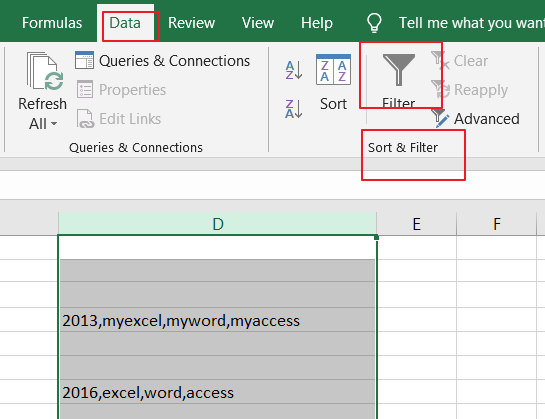#6 click the Filter icon in Cell D1, and uncheck `Blanks` checkbox, and then click `Ok` button.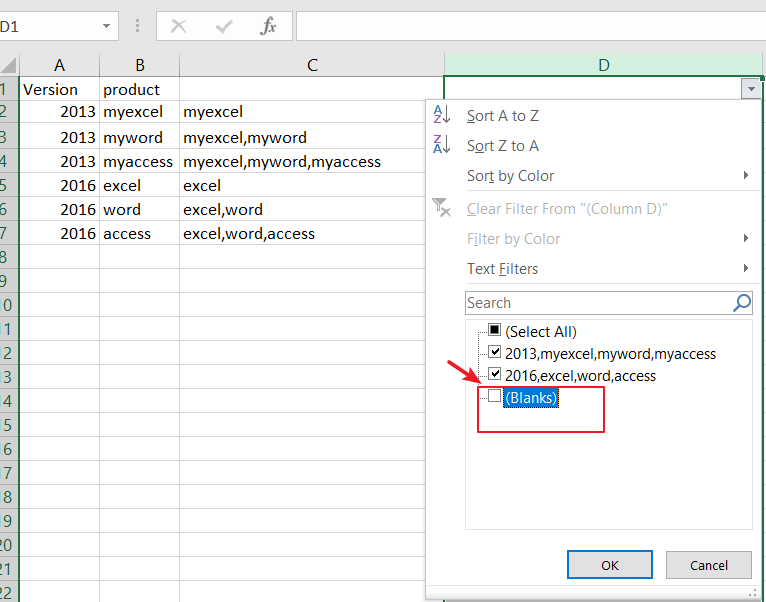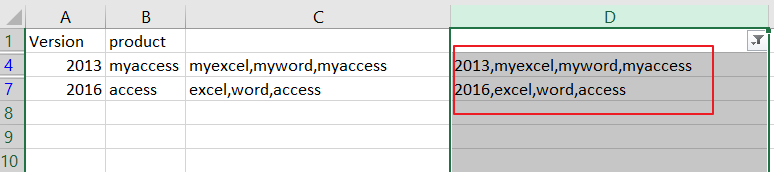### Concatenate Cells If Same Value Exists in Another Column using VBA

You can also use an Excel VBA Macro to concatenate cells in Range B1:B6 if same values exists in range A1:A6. Just do the following steps:

Step1: open your excel workbook and then click on “`Visual Basic`” command under `DEVELOPER` Tab, or just press “`ALT+F11`” shortcut.Step2: then the “`Visual Basic Editor`” window will appear.

Step3: click “`Insert`” ->”`Module`” to create a new module.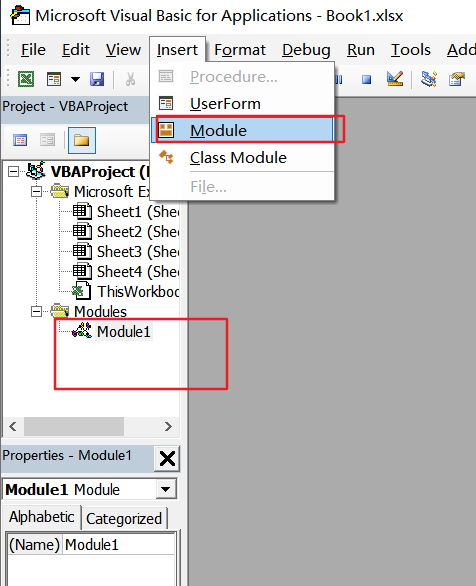Step4: paste the below VBA code  into the code window. Then clicking “`Save`” button.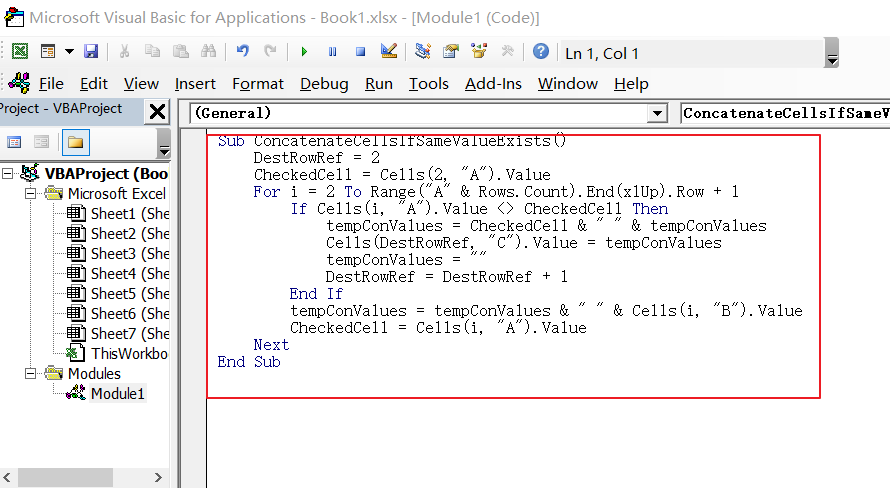```Sub ConcatenateCellsIfSameValueExists()
DestRowRef = 2
CheckedCell = Cells(2, "A").Value
For i = 2 To Range("A" & Rows.Count).End(xlUp).Row + 1
If Cells(i, "A").Value <> CheckedCell Then
tempConValues = CheckedCell & " " & tempConValues
Cells(DestRowRef, "C").Value = tempConValues
tempConValues = ""
DestRowRef = DestRowRef + 1
End If
tempConValues = tempConValues & " " & Cells(i, "B").Value
CheckedCell = Cells(i, "A").Value
Next
End Sub```

Note: this VBA Macro will compare cell values in column A, and then concatenate cell values in Column B, and palce the concatenated values into column C, and started from Cell C2.

Step5: back to the current worksheet, click on `Macros` button under `Code` group. then click Run button.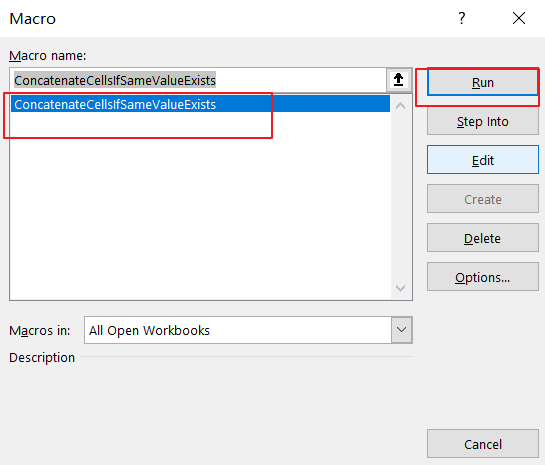Step6: let’s see the last result: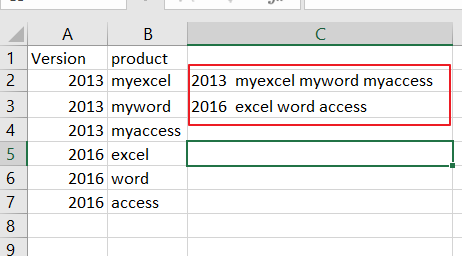### Related Functions

• Excel IF function
The Excel IF function perform a logical test to return one value if the condition is TRUE and return another value if the condition is FALSE. The IF function is a build-in function in Microsoft Excel and it is categorized as a Logical Function.The syntax of the IF function is as below:= IF (condition, [true_value], [false_value])….